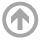Spring 2020 :: Mathematics Department :: USNA
Mathematics Department

# Operator Algebras and Dynamics Seminars

## Spring 2020

All talks are from 3:45-4:45 p.m. in the Seminar room, unless otherwise specified.

• Apr
29
• [Postponed]
Cesar Silva
Williams College
Time: 03:45 PM
• Apr
20
• [Postponed] Superstability and Finite-Time Extinction for C0-Semigroups
Darren Creutz
USNA
Time: 03:45 PM

#### View Abstract

[Joint work with M. Mazo, Jr.] Semigroups of operators arise naturally in many areas, particularly Control Theory and PDEs. Specifically, a family of operators {T(t) : t >= 0} such that T(t+s) = T(t)T(s) and t |--> T(t) is strongly continuous is a C0-semigroup and can always be realized as T(t) = exp(At) for some operator A, the infinitesimal generator. A C0-semigroup is (exponentially) stable when for some M, omega > 0 and all t >= 0, ||T(t)|| <= M exp(-omega t) and the maximal such omega is the stability index. Stable semigroups are the most useful and many equivalent characterizations (some of which I will mention) exist. Control theorists introduced the notion of superstable semigroups (circa 1990s), those where the stability index is infinite, having realized that finite-time extinction (T(t) = 0 eventually) occurred in many physical systems and clearly implies superstability. A natural question is whether superstability implies finite-time extinction. Balakrishnan (circa 2005) asked whether there exists a superstable semigroup with infinitesimal generator being a differential ("physical") operator that does not go extinct in finite time. We answer this question in the affirmative by introducing a variety of new machinery for studying the resolvent sets of the semigroup.
• Apr
13
• [Postponed]
Sayomi Kamimoto
Location: George Washington University
Time: 03:45 AM
• Mar
30
• [Postponed]